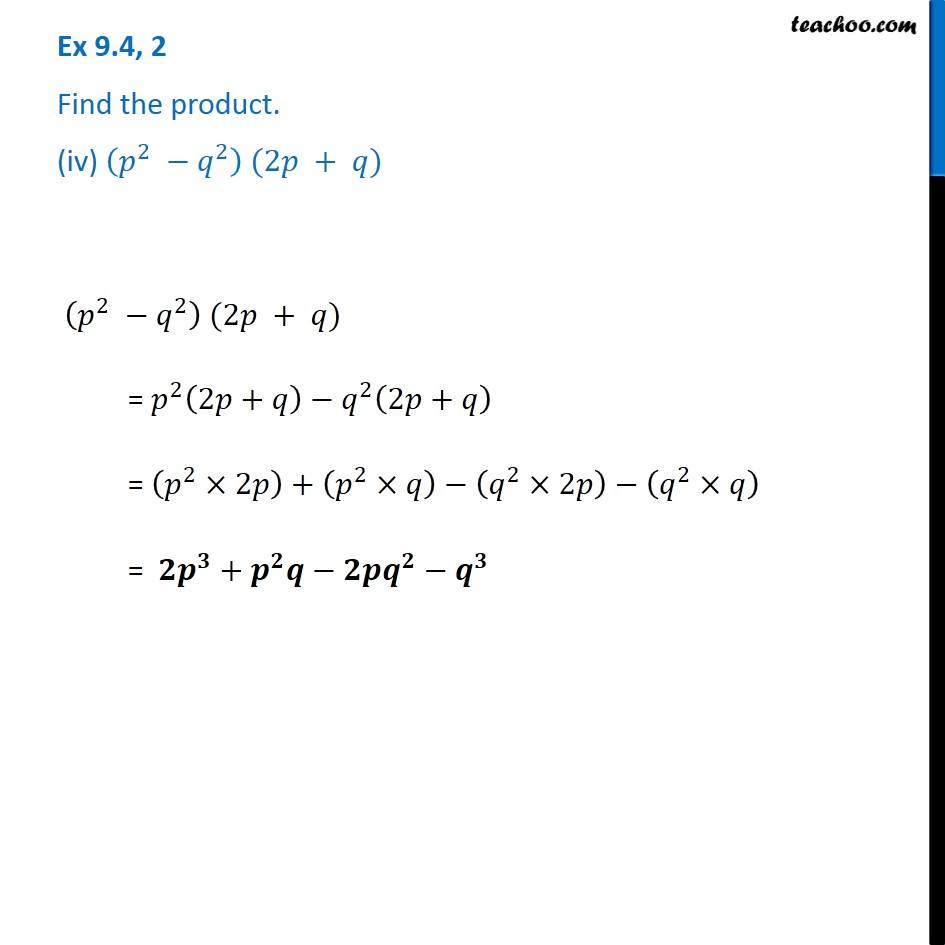Subscribe to our Youtube Channel - https://you.tube/teachoo

1. Chapter 9 Class 8 Algebraic Expressions and Identities
2. Concept wise
3. Multiplication of Polynoimals by Polynomials

Transcript

Ex 9.4, 2 Find the product. (iv) (𝑝^2 −𝑞^2 ) (2𝑝 + 𝑞) (𝑝^2 −𝑞^2 ) (2𝑝 + 𝑞) = 𝑝^2 (2𝑝+𝑞)−𝑞^2 (2𝑝+𝑞) = (𝑝^2×2𝑝)+(𝑝^2×𝑞)−(𝑞^2×2𝑝)−(𝑞^2×𝑞) = 𝟐𝒑^𝟑+𝒑^𝟐 𝒒−𝟐𝒑𝒒^𝟐−𝒒^𝟑

Multiplication of Polynoimals by Polynomials